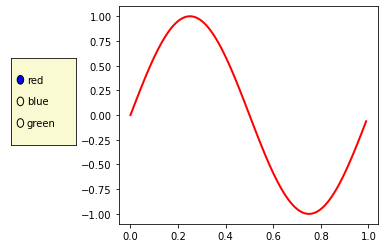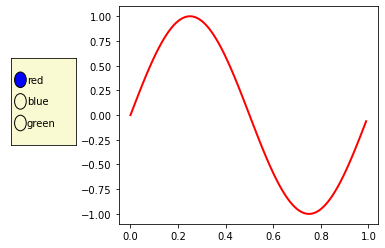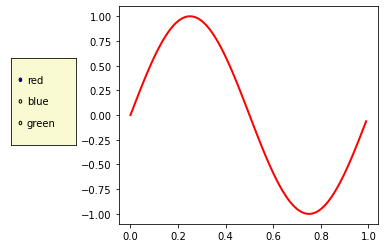Related Articles
How to resize Matplotlib RadioButtons?
• Last Updated : 03 Mar, 2021

Matplotlib is an amazing visualization library in Python for 2D plots of arrays. Matplotlib is a multi-platform data visualization library built on NumPy arrays and designed to work with the broader SciPy stack.

Radio buttons are the most commonly used widgets in visualization. Radio buttons let us choose between multiple options in a visualization. In this case, the buttons let the user choose one of the three different colors for the sine wave to be shown in the plot.

This is the simple visualization of the radio buttons.

## Python3

 `# import modules``import` `numpy as np``import` `matplotlib.pyplot as plt``from` `matplotlib.widgets ``import` `RadioButtons`` ` `# creating an array starting from ``# 0 to 1 with step size 0.01``t ``=` `np.arange(``0.0``, ``1.0``, ``0.01``)`` ` `# the values of sin values of t``s0 ``=` `np.sin(``2``*``np.pi``*``t)`` ` `# depict visuliation``fig, ax ``=` `plt.subplots()``l, ``=` `ax.plot(t, s0, lw``=``2``, color``=``'red'``)``plt.subplots_adjust(left``=``0.3``)`` ` `# adjust radio buttons``axcolor ``=` `'lightgoldenrodyellow'``rax ``=` `plt.axes([``0.05``, ``0.4``, ``0.15``, ``0.30``], ``               ``facecolor``=``axcolor)`` ` `radio ``=` `RadioButtons(rax, (``'red'``, ``'blue'``, ``'green'``))`` ` `plt.show()`

Output:In the above code, plt.axes() adds axes to the current figure and makes it the current axes. The first argument is 4-tuple of floats for the box  = [left, bottom, width, height]. The second parameter is **kwargs. This method also takes the keyword arguments for the returned axes class. It can be facecolor, figure, etc. In method RadioButtons(), the first argument is the axes created above and the second argument is the tuple of the labels we want.

### Resizing the radius of the circles in the Radio Button

The default size of the circle is 0.05. We add a simple line as shown below to change the radius. If the radius of the circle is greater than 0.05 say 0.09.

## Python3

 `# import module``import` `numpy as np``import` `matplotlib.pyplot as plt``from` `matplotlib.widgets ``import` `RadioButtons`` ` `# creating an array starting from``# 0 to 1 with step size 0.01``t ``=` `np.arange(``0.0``, ``1.0``, ``0.01``)`` ` `# the values of sin values of t``s0 ``=` `np.sin(``2``*``np.pi``*``t)`` ` `# depict visualiiation``fig, ax ``=` `plt.subplots()``l, ``=` `ax.plot(t, s0, lw``=``2``, color``=``'red'``)``plt.subplots_adjust(left``=``0.3``)`` ` `# adjust radiobuttons``axcolor ``=` `'lightgoldenrodyellow'``rax ``=` `plt.axes([``0.05``, ``0.4``, ``0.15``, ``0.30``], ``               ``facecolor``=``axcolor)``radio ``=` `RadioButtons(rax, (``'red'``, ``'blue'``, ``'green'``))`` ` `# adjust radius here. The default is 0.05``for` `circle ``in` `radio.circles:``    ``circle.set_radius(``0.09``)`

Output:Here is another example where the radius of the circle is less than 0.05 say 0.02

## Python3

 `# import module``import` `numpy as np``import` `matplotlib.pyplot as plt``from` `matplotlib.widgets ``import` `RadioButtons`` ` `# creating an array starting from``# 0 to 1 with step size 0.01``t ``=` `np.arange(``0.0``, ``1.0``, ``0.01``)`` ` `# the values of sin values of t``s0 ``=` `np.sin(``2``*``np.pi``*``t)`` ` `# depict visualiation``fig, ax ``=` `plt.subplots()``l, ``=` `ax.plot(t, s0, lw``=``2``, color``=``'red'``)``plt.subplots_adjust(left``=``0.3``)`` ` `# adjust radiobuttons``axcolor ``=` `'lightgoldenrodyellow'``rax ``=` `plt.axes([``0.05``, ``0.4``, ``0.15``, ``0.30``],``               ``facecolor``=``axcolor)`` ` `radio ``=` `RadioButtons(rax, (``'red'``, ``'blue'``, ``'green'``))`` ` `# adjust radius here. The default is 0.05``for` `circle ``in` `radio.circles:``    ``circle.set_radius(``0.02``)`

Output:Attention geek! Strengthen your foundations with the Python Programming Foundation Course and learn the basics.

To begin with, your interview preparations Enhance your Data Structures concepts with the Python DS Course.

My Personal Notes arrow_drop_up